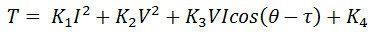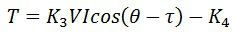# Universal Relay Torque Equation

The universal torque equation explains the working of an electrical relay. The relay has some arrangement of electromagnetic. These electromagnetic consist current and voltage windings. The current through the winding produces magnetic flux. And the torque is produced by the interaction of the flux of the same winding or between the flux of both the windings.If both the current and voltage windings are used, the torque developed by the interaction between the fluxes is given by the equationWhere θ is the angle between V and I and the τ is the relay maximum torque angle.

If the relay has current, voltage and the torque angle, the torque will be developed, and it will be given aswhere K1, K2, K3  are the tap setting or constant of V and I. The K4 is the mechanical restraint due to spring or gravity.

The operating characteristic of all types of relays is obtained by adding and subtracting all the other constants and letting others be zero or by adding other similar terms.

For example – In over current relay the K2 = K3= 0 because of the absence of the voltage windings. The torque equation becomesThe negative sign attributes to K4 because the spring produces restraining torque.

Similarly, for directional relay K1 = K2 = 0 and the developed torque will be given as